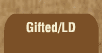Related links at Resource Room:

Mathematics and Dyslexia

Tips for Learning the Times Tables

Tools for the Times Tables & Workbook

Home > Math > times tables practice > zeroes, ones and tens

## Tens, Ones, and Zeroes

1. 8 x 0=
2. 1 x 3=
3. 10 x 2=
4. 0 x 0=
5. 10 x 10=
6. 9 x 1=
7. 4 x 0=
8. 4 x 1=
9. 10 x 4=
10. 1 x 7=
11. 10 x 0=
12. 2 x 1=
13. 6 x 0=
14. 0 x 1=
15. 8 x 10=
16. 1 x 5=
17. 10 x 6=
18. 7 x 0=
19. 1 x 8=
20. 10 x 3=
21. 9 x 10=
22. 5 x 0=
23. 10 x 7=
24. 0 x 2=
25. 1 x 6=
26. 8 x 10=
27. 9 x 0=
28. 0 x 3=
29. 10 x 5=
30. 1 x 1=
31. 1 x 9=
32. 7 x 10=
33. 53 x 10=
34. 19 x 0=
35. 1 x 37=
36. Bonus! 7 x 6=

copyright © 1998-2003 Susan Jones, Resource Room. All Rights Reserved.